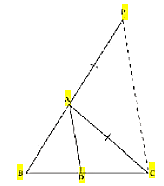Guru

# In Figure, D is a point on side BC of ∆ ABC such that BD/CD = AB/AC. Prove that AD is the bisector of ∠ BAC. Q.9

• 0

Hello, sir give me the best way to solve the problem of Triangles chapter of class 10th of exercise 6.6 of math sir please give me the easy solution of this question In Figure, D is a point on side BC of ∆ ABC such that BD/CD = AB/AC. Prove that AD is the bisector of ∠ BAC.

Share

1. In the given figure, let us extend BA to P such that;

AP = AC.

Now join PC.Given, BD/CD = AB/AC

⇒ BD/CD = AP/AC

By using the converse of basic proportionality theorem, we get,

∠BAD = ∠APC (Corresponding angles) ……………….. (i)

And, ∠DAC = ∠ACP (Alternate interior angles) …….… (ii)

By the new figure, we have;

AP = AC

⇒ ∠APC = ∠ACP ……………………. (iii)

On comparing equations (i), (ii), and (iii), we get,